## Lecture 9 – Data 100, Fall 2020¶

by Suraj Rampure, updates by Fernando Pérez.

In :
import numpy as np
import pandas as pd
import matplotlib.pyplot as plt
import seaborn as sns

In :
births = pd.read_csv('baby.csv')

In :
births.head()

Out:
Birth Weight Gestational Days Maternal Age Maternal Height Maternal Pregnancy Weight Maternal Smoker
0 120 284 27 62 100 False
1 113 282 33 64 135 False
2 128 279 28 64 115 True
3 108 282 23 67 125 True
4 136 286 25 62 93 False
In :
births.shape

Out:
(1174, 6)

## Bar Plots¶

We often use bar plots to display distributions of a categorical variable:

In :
births['Maternal Smoker'].value_counts()

Out:
False    715
True     459
Name: Maternal Smoker, dtype: int64
In :
births['Maternal Smoker'].value_counts().plot(kind = 'bar');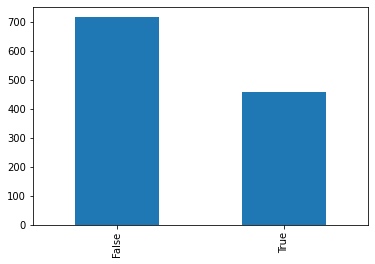Note: putting a semicolon after a plot call hides all of the unnecessary text that comes after it (the <matplotlib.axes_....>).

In :
sns.countplot(x=births['Maternal Smoker']);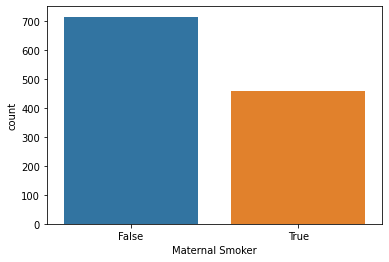But we can also use them to display a numerical variable that has been measured on individuals in different categories.

In :
# These are made up!
majors = ['Data Science', 'History', 'Biology', 'Business']
gpas = [3.35, 3.20, 2.98, 3.51]

In :
# What if we change bar to barh?
plt.bar(majors, gpas);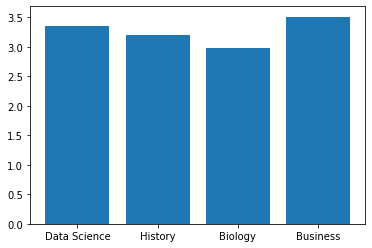In :
sns.barplot(x=majors, y=gpas);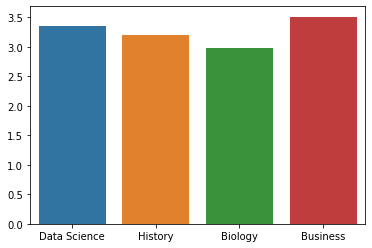## Rug plots¶

Used for visualizing a single quantitative variable. Rug plots show us each and every value.

In :
bweights = births["Birth Weight"]

In :
bweights

Out:
0       120
1       113
2       128
3       108
4       136
...
1169    113
1170    128
1171    130
1172    125
1173    117
Name: Birth Weight, Length: 1174, dtype: int64
In :
sns.rugplot(bweights);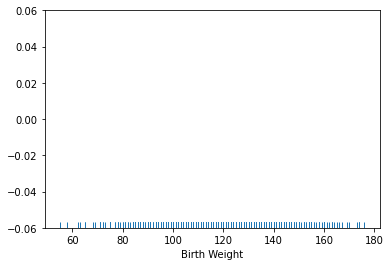## Histograms¶

Our old friend!

In :
# By default, you get some arbitrary bins. We often like to pick our own.
plt.hist(bweights);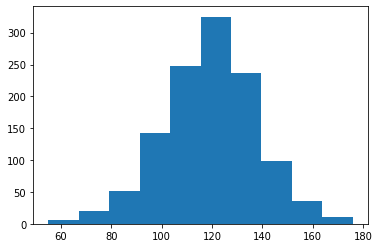In :
min(bweights), max(bweights)

Out:
(55, 176)
In :
bw_bins = range(50, 200, 5)

In :
plt.hist(bweights, bins=bw_bins, ec='w');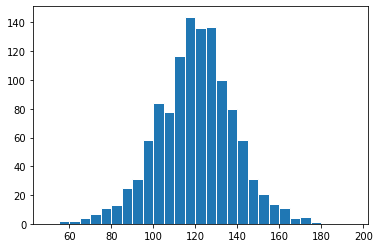The above plot shows counts, if we want to see a distribution we can use the density keyword:

In :
plt.hist(bweights, density=True, bins=bw_bins, ec='w');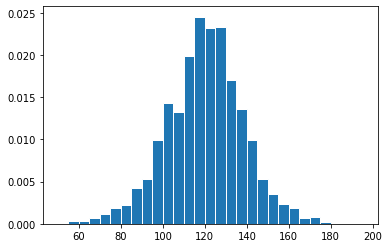In :
# alternative way of getting this plot
bweights.plot(kind = 'hist', density=True, bins=bw_bins, ec='w');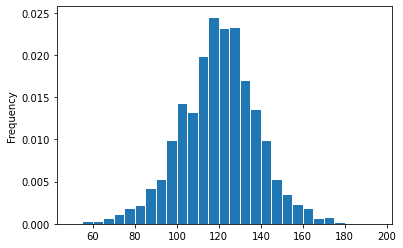Increasing bin width loses granularity, but this may be fine for our purposes.

In :
plt.hist(bweights, bins = np.arange(50, 200, 20), density=True, ec='w');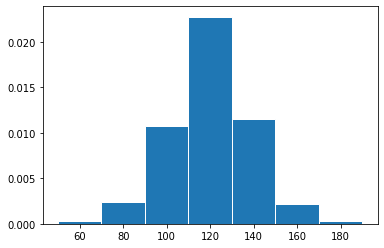The bin widths don't all need to be the same!

In :
plt.hist(bweights, bins = [50, 100, 120, 140, 200], density=True, ec='w');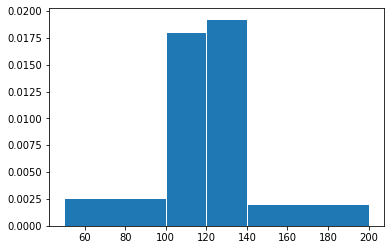## Density Curves¶

In :
sns.kdeplot(bweights);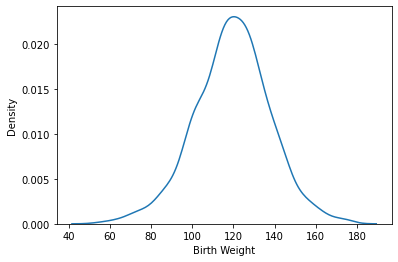Seaborn has several related functions for plotting distributions: kdeplot, histplot, rugplot and displot. The latter is more generic but uses the others under the hood:

In :
sns.histplot(bweights, kde=True);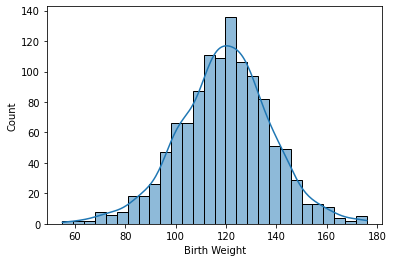Can even show a rugplot with it!

In :
sns.displot(bweights, kde=True, rug=True);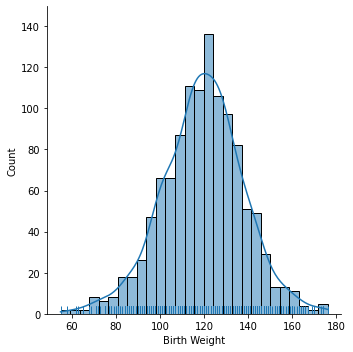displot is quite flexible, so instead of a histogram we can ask it, for example, to show the density curve and rugplot only:

In :
sns.displot(bweights, kind='kde', rug=True);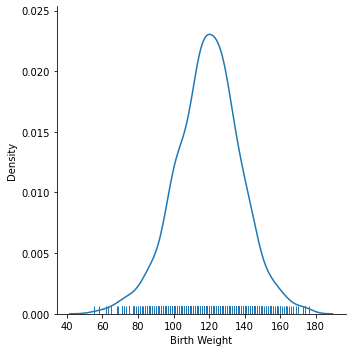## Box Plots¶

In :
plt.figure(figsize = (3, 6))
sns.boxplot(y=bweights);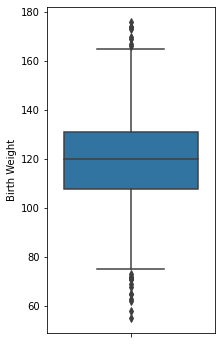In :
q1 = np.percentile(bweights, 25)
q2 = np.percentile(bweights, 50)
q3 = np.percentile(bweights, 75)
iqr = q3 - q1
whisk1 = q1 - 1.5*iqr
whisk2 = q3 + 1.5*iqr

whisk1, q1, q2, q3, whisk2

Out:
(73.5, 108.0, 120.0, 131.0, 165.5)

## Violin Plots¶

In :
plt.figure(figsize = (3, 6))
sns.violinplot(y=bweights);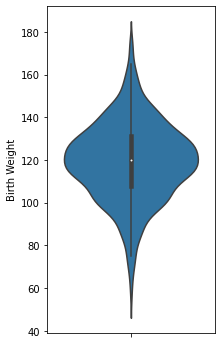## Overlaid Histograms and Density Curves¶

In :
births.head()

Out:
Birth Weight Gestational Days Maternal Age Maternal Height Maternal Pregnancy Weight Maternal Smoker
0 120 284 27 62 100 False
1 113 282 33 64 135 False
2 128 279 28 64 115 True
3 108 282 23 67 125 True
4 136 286 25 62 93 False
In :
sm_bweights = births[births['Maternal Smoker'] == True]['Birth Weight']
nsm_bweights = births[births['Maternal Smoker'] == False]['Birth Weight']

In :
sns.histplot(nsm_bweights, bins=bw_bins, kde=True, stat='density', label='non smoker', ec='w');
sns.histplot(sm_bweights, bins=bw_bins, kde=True, stat='density', label='smoker', color='orange', ec='w');
plt.legend();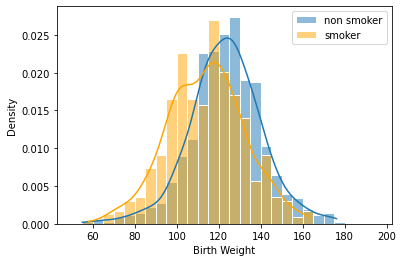## Side by side box plots and violin plots¶

In :
plt.figure(figsize=(5, 8))
sns.boxplot(data=births, x = 'Maternal Smoker', y = 'Birth Weight');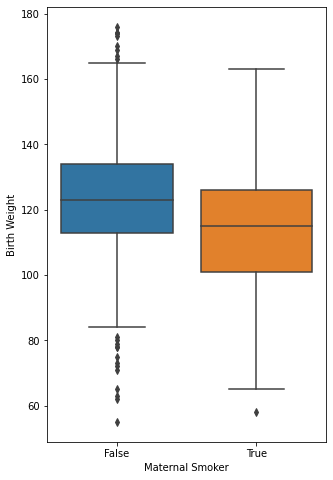In :
plt.figure(figsize=(5, 8))
sns.violinplot(data=births, x = 'Maternal Smoker', y = 'Birth Weight');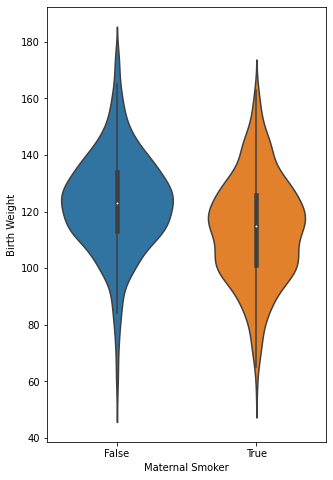A less fancy version of the above two plots:

In :
two_distributions = [nsm_bweights.values, sm_bweights.values]
groups = ['non-smokers', 'smokers']

In :
plt.boxplot(two_distributions, labels=groups);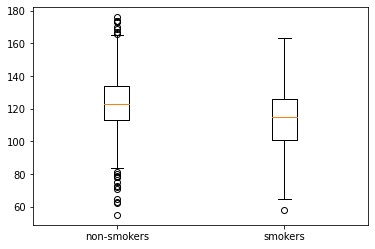In :
plt.violinplot(two_distributions);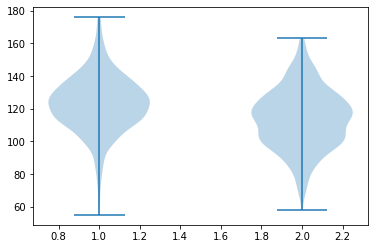## Scatter plots¶

In :
births.head()

Out:
Birth Weight Gestational Days Maternal Age Maternal Height Maternal Pregnancy Weight Maternal Smoker
0 120 284 27 62 100 False
1 113 282 33 64 135 False
2 128 279 28 64 115 True
3 108 282 23 67 125 True
4 136 286 25 62 93 False
In :
plt.scatter(births['Maternal Height'], births['Birth Weight']);
plt.xlabel('Maternal Height')
plt.ylabel('Birth Weight');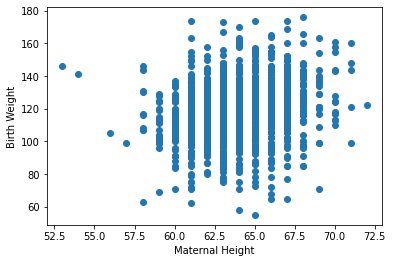In :
plt.scatter(data=births, x='Maternal Height', y='Birth Weight');
plt.xlabel('Maternal Height')
plt.ylabel('Birth Weight');In :
sns.scatterplot(data = births, x = 'Maternal Height', y = 'Birth Weight', hue = 'Maternal Smoker');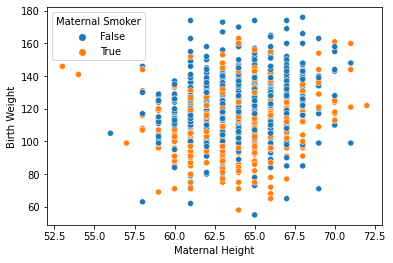In :
sns.lmplot(data = births, x = 'Maternal Height', y = 'Birth Weight', ci=False, hue='Maternal Smoker');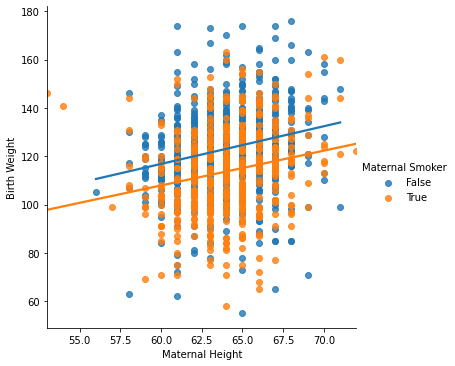In :
sns.jointplot(data = births, x = 'Maternal Height', y = 'Birth Weight');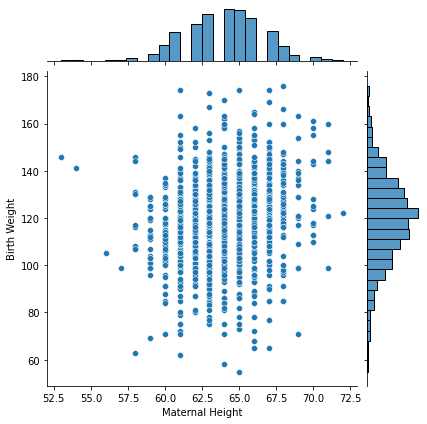In :
sns.jointplot(data = births, x = 'Maternal Height', y = 'Birth Weight', hue='Maternal Smoker');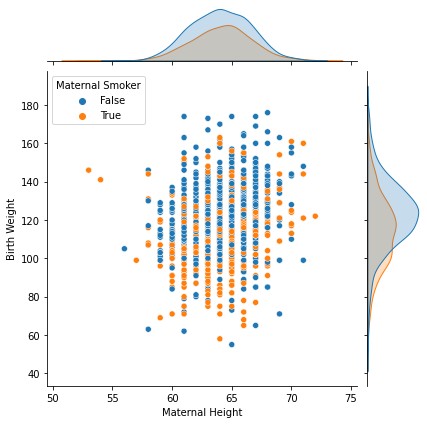## Hex plots and contour plots¶

In :
sns.jointplot(data = births, x = 'Maternal Height', y = 'Birth Weight', kind='hex');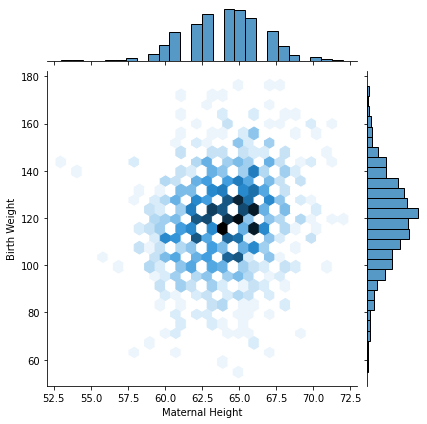In :
sns.jointplot(data = births, x = 'Maternal Height', y = 'Birth Weight', kind='kde', fill=True);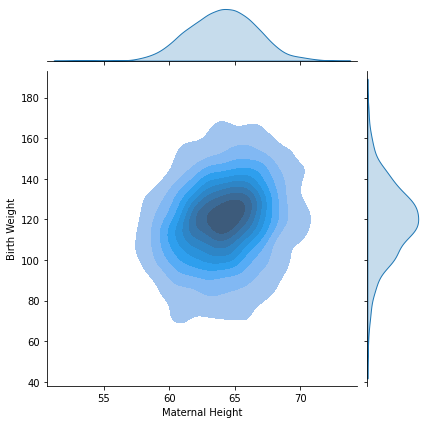In :
sns.jointplot(data = births, x = 'Maternal Height', y = 'Birth Weight', kind='kde', hue='Maternal Smoker');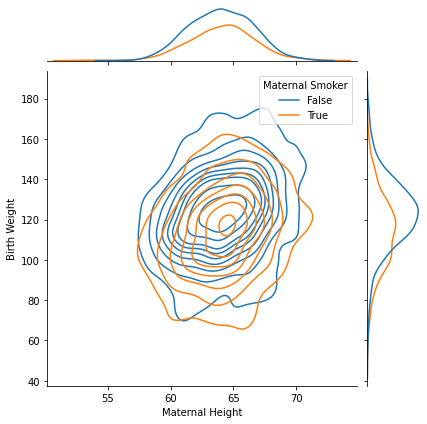## Bonus¶

Calling .plot() results in weird things!

In :
births.plot();